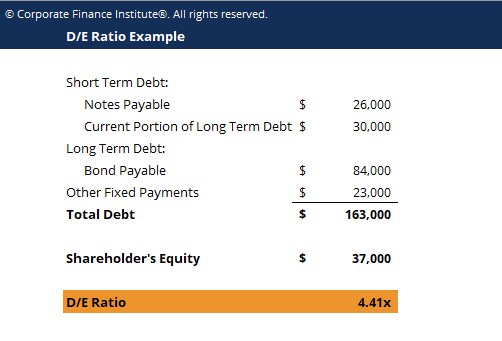# Debt Equity Ratio Template

## Debt Equity Ratio Template

This debt equity ratio template shows you how to calculate D/E ratio given the amounts of short-term and long-term debt and shareholder’s equity.

This is what the debt equity ratio template looks like:### Download the Free Template

Enter your name and email in the form below and download the free template now!

### Debt Equity Ratio Template

Download the free Excel template now to advance your finance knowledge!

The Debt to Equity ratio (also called the “debt-equity ratio”, “risk ratio” or “gearing”), is a leverage ratio that calculates the weight of total debt and financial liabilities against the total shareholder’s equity. Unlike the debt-assets ratio which uses total assets as a denominator, the debt to equity ratio uses total equity. This ratio highlights how a company’s capital structure is tilted either toward debt or equity financing.

### Debt to Equity ratio formula

Short formula:

Debt to Equity Ratio = Total Debt / Shareholders’ Equity

Long formula:

Debt to Equity Ratio = (short term debt + long term debt + fixed payment obligations) / Sharehoders’ Equity

A high debt-equity ratio can be good when a firm can easily service its debt obligations (through cash flow) and is using the leverage to increase equity returns.

### More Free Templates

For more resources, check out our business templates library to download numerous free Excel modeling, PowerPoint presentation and Word document templates.

• Excel Modeling Templates
• PowerPoint Presentation Templates
• Transaction Document Templates

### Financial Analyst Certification

Become a certified Financial Modeling and Valuation Analyst (FMVA)® by completing CFI’s online financial modeling classes and training program!